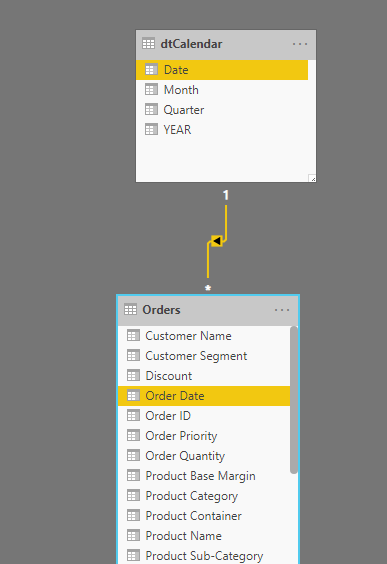Search

# Moving Average using DAXLet us consider a data set of sales. The objective is to analyze daily sales volume. If we simply build a report that plots the sales amount sliced by day, the result is difficult to decipher.

Following is the time series of day-wise total sales:

`Total Sales = SUM(Orders[Sales])`Daily sales volume over 5 years

The graph above shows high variations in total sales value with a horizontal trend pattern.

In statistical data modeling, a common approach is to calculate the average over a certain period higher than just the day level, especially when the time series shows a horizontal pattern over a period. It is also known as Moving Average.

## What is a moving average?

The moving averages method uses the average of the most recent k data values in the time series. We call it moving because every time a new observation becomes available for the time series, it replaces the oldest observation in the equation with the latest value and calculates a new average. Thus, the periods over which the average is calculated changes, or moves, with each ensuing period.

Following is an example of 3 periods moving average (k = 3)## Calculating Moving Average using DAX

The objective here is to calculate the moving average of the last 30 days. So, k = 30

Experts recommend creating at least one calendar table in the data model. In the current model, I have created a calendar table and established the relationship:You may refer to the following article on Calendar Tables.

Then, create a measure with the following code:

```Avg Sales (MA 30 days) =

//Selecting the date in the range
VAR _LastDate =
MAX ( dtCalendar[Date] )

//Defining the duration to be considered for average calculation(k)
VAR _Duration = 30

//Filtering the Calendar Table for the defined range
VAR _CalculationPeriod =
FILTER (
ALL ( dtCalendar ),  --Removing any external filters context applied
AND (
dtCalendar[Date] > _LastDate - _Duration,  --the range start date
dtCalendar[Date] <= _LastDate  --the range end date
)
)

//Calculating the Moving Average
VAR _MovingAverage =
IF (
COUNTROWS ( _CalculationPeriod ) >= _Duration, --Condition to check minimum number of days for the calculation.
CALCULATE (
AVERAGEX(dtCalendar,[Total Sales]),  --Calculating average of the total sales amount
_CalculationPeriod
)
)
RETURN
_MovingAverage```

The formula first determines the last visible date; in the visual, because the filter context set by the visual is at the date level, it returns the selected date (VAR _LastDate)

Then it creates a set of all the dates between the last date and the last date minus 30 days (VAR _CalculationPeriod).

Finally, the last step is to use this period as a filter in CALCULATE, so that the final AVERAGEX iterates over the 30 days, computing the average of the daily sales.

Following graph presents the output:The Moving Average line is smoother than the daily sales, which simplifies the trend analysis process.

@imVivRan